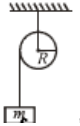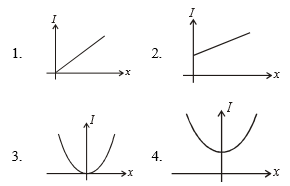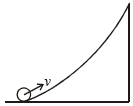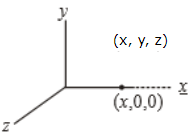A wheel is rolling along a horizontal ground with a speed o 10 m/s. The magnitude of the velocity of the points at the extremities of the horizontal diameter of the wheel is equal to

(1)

(2) 10 m/s

(3)

(4)

Concept Questions :-

Rolling motion
High Yielding Test Series + Question Bank - NEET 2020

Difficulty Level:

A projectile of mass 50 kg is shot vertically upwards with an initial velocity of 100ms-1. After 5 seconds it explodes into two fragments, one of which having mass 20 kg, travels vertically up with a velocity of 150 ms-1. The velocity of the other fragment at that instant is -          [Take g= 9.8 m/${s}^{2}$]

1.  100 ms-1

2.  150 ms-1

3.  -150 ms-1

4.  -15 ms-1

Concept Questions :-

Linear momentum
High Yielding Test Series + Question Bank - NEET 2020

Difficulty Level:

A sphere of mass m moving with constant velocity hits another sphere of the same mass at rest. If e is the coefficient of restitution. The ratio of their velocities after the collision is

1.  1 + e

2.

3.

4.

Concept Questions :-

Linear momentum
High Yielding Test Series + Question Bank - NEET 2020

Difficulty Level:

Concept Questions :-

Angular momentum
High Yielding Test Series + Question Bank - NEET 2020

Difficulty Level:

A mass M is moving with constant velocity parallel to the X-axis. Its angular momentum w.r.t. origin is:

1.  Zero

2.  Remains constant

3.  goes on increasing

4.  goes on decreasing

Concept Questions :-

Angular momentum
High Yielding Test Series + Question Bank - NEET 2020

Difficulty Level:

A pulley of mass m & radius R is free to rotate about its axis which is horizontal. A string is wound around it and mass m is attached to its free end. When mass m falls through a distance h, its speed at that instant is:1.  proportional to R

2.  proportional to 1/R

3.  proportional to 1/R2

4.  independent of R

Concept Questions :-

Torque
High Yielding Test Series + Question Bank - NEET 2020

Difficulty Level:Concept Questions :-

Moment of inertia
High Yielding Test Series + Question Bank - NEET 2020

Difficulty Level:

A particle is rotating under the central force, in which one of the following quantities is constant:

1.  torque

2.  angular momentum

3.  linear momentum

4.  none of these

Concept Questions :-

Angular momentum
High Yielding Test Series + Question Bank - NEET 2020

Difficulty Level:

A small object of uniform density rolls up a curved surface with an initial velocity v. It is reached upto a maximum height of $\frac{3{\mathrm{v}}^{2}}{4\mathrm{g}}$ w.r.t. the initial position. The object is:1.  Ring

2.  Solid sphere

3.  Hollow sphere

4.  Disc

Concept Questions :-

Rolling motion
High Yielding Test Series + Question Bank - NEET 2020

Difficulty Level:

A point is at (x, y, z). What is its moment of inertia about x-axis:1.  $\mathrm{m}\left({\mathrm{x}}^{2}+{\mathrm{y}}^{2}+{\mathrm{z}}^{2}\right)$

2.  $\mathrm{m}\left({\mathrm{x}}^{2}+{\mathrm{y}}^{2}\right)$

3.  $\mathrm{m}\left({\mathrm{y}}^{2}+{\mathrm{z}}^{2}\right)$

4.  $\mathrm{m}\left({\mathrm{x}}^{2}+{\mathrm{z}}^{2}\right)$

Concept Questions :-

Moment of inertia